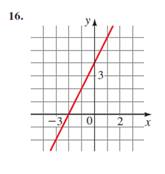# Equation of the line whose graph is sketched.### Precalculus: Mathematics for Calcu...

6th Edition
Stewart + 5 others
Publisher: Cengage Learning
ISBN: 9780840068071### Precalculus: Mathematics for Calcu...

6th Edition
Stewart + 5 others
Publisher: Cengage Learning
ISBN: 9780840068071

#### Solutions

Chapter 1.10, Problem 16E
To determine

## Equation of the line whose graph is sketched.

Expert Solution

4x2y+8=0

### Explanation of Solution

Given information:Formula used:

Intercept form of line= xa+yb=1

Where (x,y) is a point on line and a and b are intercept cut by line on x-axis and y-axis .

As shown in figure, line cuts intercept of -2 and 4 on x-axis and y-axis respectively.

xa+yb=1

a=2b=4

x2+y4=14x+(2)y(2)4=14x2y=(8)4x2y+8=0

### Have a homework question?

Subscribe to bartleby learn! Ask subject matter experts 30 homework questions each month. Plus, you’ll have access to millions of step-by-step textbook answers!